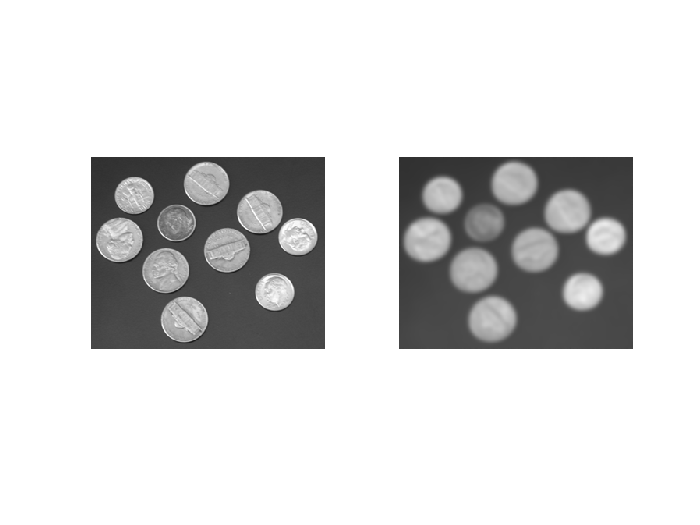# PDEs: Solution of the 2D Heat Equation using Finite Differences

This is an example of the numerical solution of a Partial Differential Equation using the Finite Difference Method. In this case applied to the Heat equation.
To approximate the derivative of a function in a point, we use the finite difference schemes.

## 1D Finite Differences

One can choose different schemes depending on the final wanted precission.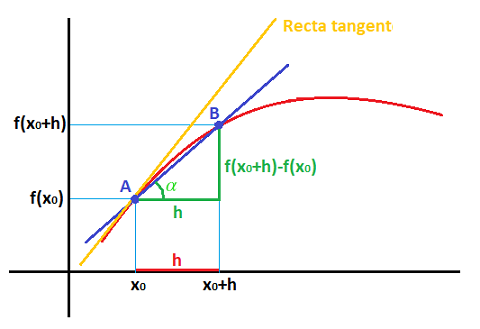• Forward Differences: error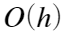• Central Differences: error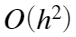• Second derivative. Central Differences: error## Partial Derivatives: 2D Finite Differences

For a funtion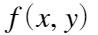the second order partial derivatives can be approximated by: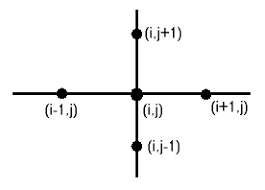## 2D Regular Domains

To apply finite differences to a rectangular domain, it must be divided in equal spaced points. One example of rectangular 2D domain can be an image or a photograph. We are used to work with the pixel decomposition of an image.
To load an image in Matlab is very easy, you can use:
Aorig = imread('coins.png'); %read the original image
imshow(Aorig); %show the original image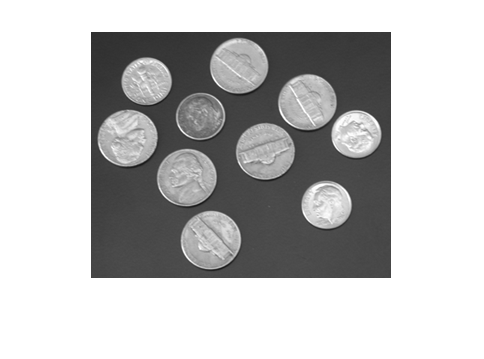A = im2double(Aorig); % By default pixel values are 8 bits integer numbers,
% to do some algebra computations we better use double float numbers

## Application: Image blur using the heat equation

The PDE modeling temperature distribution along the time iswhere the function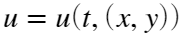depens on time and space variables.
To simulate the time evolution of the temperatures, we need an initial temperature distribution. For that we can take the original pixel values (in gray scale) and assimilate temperature to the pixel intensity value. This way, white pixels will be the hotter and dark ones the colder.
Matlab allow you to visualize these temperatures creating a 3D plot using the following instructionsm(you can rotate the 3D plot using the circular icon on the Matlab window and moving the mouse).
figure(2) %Initial temperature distribution
x=1:size(A,2);
y=1:size(A,1);
[X,Y]=meshgrid(x,y);
mesh(X,Y,A)
colormap(jet);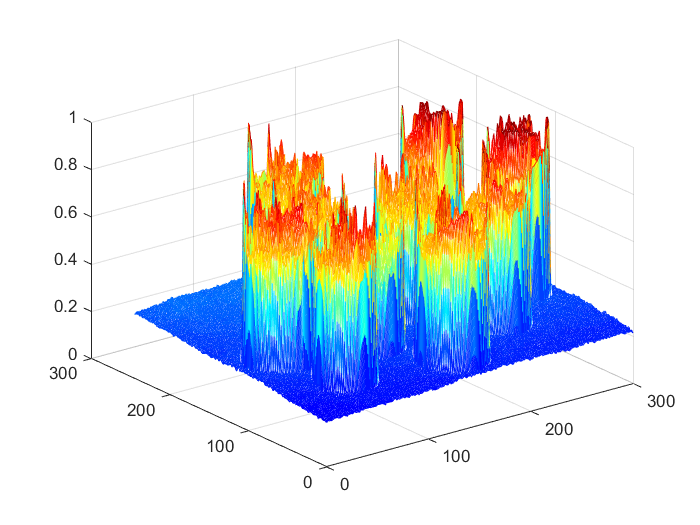## Temporal temperatures evolution

From the initial temperature distribution, we apply the heat equation on the pixels grid and we can see the effect on the temperature values. We will use a forward difference scheme for the first order temporal term and a central difference one for the second order term corresponding to derivatives with respect to the spatial variables.
The complete scheme will beIn our case the values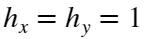(the distance is one pixel) and we take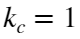and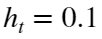The unknown variable we want to compute is the term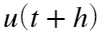(the temperature on the next time instant). Using the notation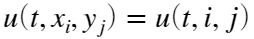we can isolate this term:In our case, the function u are the values for the pixels of image A. We simulate the evolution until time = 4.
[m,n]=size(A); %size in pixels for A.
Anext=A; %we use A=u(t) and AA=u(t+h) (at the beginnig they are the same).
ht=0.1;
hx=1;
kc=1;
r=(ht*kc)/hx^2;
tfin=4;
for t=0:ht:tfin %time advance
for j=2:n-1 %go through the pixels, but avoiding the boundary ones
for i=2:m-1
Anext(i,j)=A(i,j)+r*(A(i,j+1)+A(i+1,j)+A(i,j-1)+A(i-1,j)-4*A(i,j));
end
end
A=Anext; %update the temperature values
end

## Results after the simulation

figure(3) %final temerature distibution
imshow(A)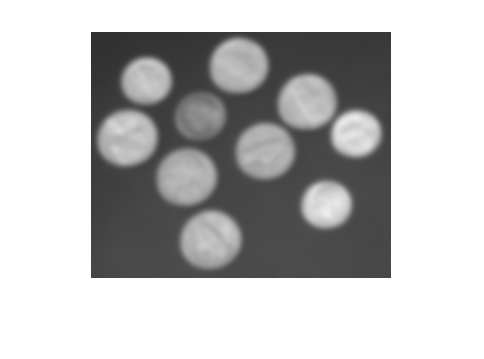mesh(X,Y,A)
colormap(jet);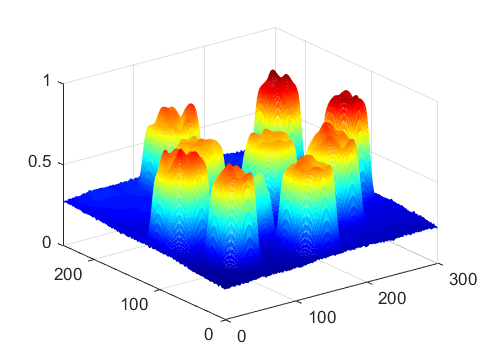## Return to images and compare with the original one

figure(4) %the final image is blurred
subplot(1,2,1)
imshow(Aorig)
subplot(1,2,2)
imshow(A)